Contact Number +90 536 226 00 45

## Understanding Current & Voltage Harmonics – 1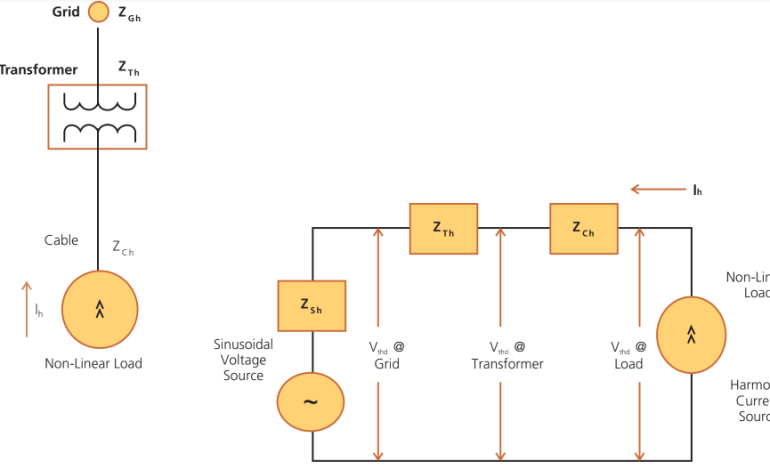Current and voltage harmonics are often used interchangeably. In most places, only harmonics are quoted and whether the values pertain to current or voltage is not mentioned. The differentiation can be done on the basis of their origin.

Understanding Total Harmonic Distortion

The current and voltage harmonics in a system are often expressed as Total Harmonic Distortion (THD). The total harmonic distortion, or THD, of a quantity, is a measurement of the harmonic distortion present and is the ratio of all harmonic components to the fundamental component. It is given by the formula as under:

 Where, is the rms value of fundamental is the rms value of  harmonic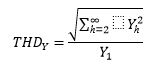Hence, current THD is the ratio of the root-mean-square value of the harmonic current to the fundamental current.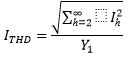Where do Current & Voltage Harmonics Originate?

Harmonics always originate as current harmonics and voltage harmonics are the results of current harmonics. Current harmonics originate because of the presence of non-linear loads like variable speed drives, inverters, UPS, television sets, PCs, semiconductors circuits, welding sets, and arc furnaces in the system. They act as harmonic current sources. The resulting current waveform can be quite complex depending on the type of load and its interaction with other components of the system.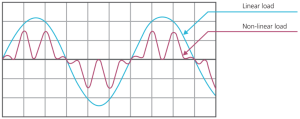Fig 1: Linear & Non-Linear Loads

The distorted current waveforms can be represented as the sum of the current waveforms of fundamental frequency and of its multiples(harmonics):Where,

c_h- Magnitude of nth order harmonics

φ_h- Phase angle of nth order harmonics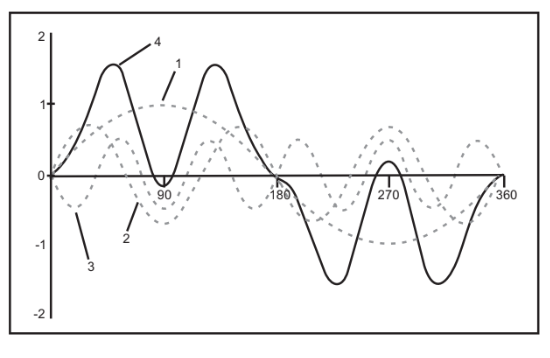Fig 2: Current waveform as sum of fundamental frequency component and its multiples

Voltage harmonics do not originate directly from non-linear loads. The current harmonics (distorted waveform) flow through system impedance (source and line impedances) and cause a harmonic voltage drop across the impedances. This will distort the supply voltage waveform. Thus voltage harmonics are generated. Long cable runs, high-impedance transformers, etc. contribute to higher source impedance and hence, higher voltage harmonics.

A typical power system has the following impedances as indicated in the line diagram: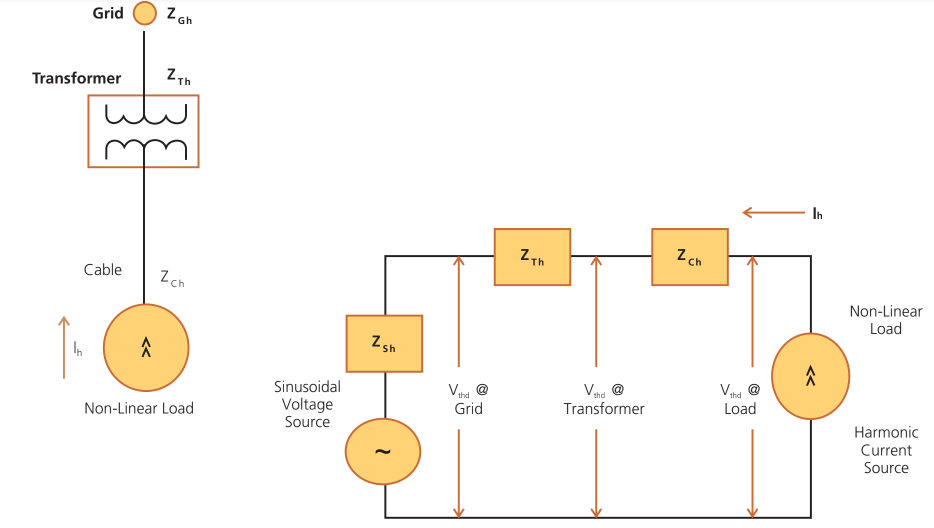Fig 3: Impedance in a power system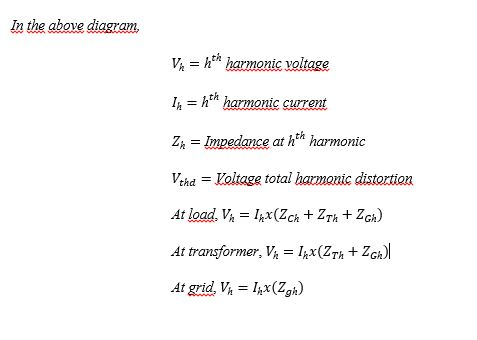Usually, grid impedances are very low and hence, the harmonic voltage distortions are also low there. However, they may be unacceptably higher on the load side as they are subjected to full system impedance there. Hence, it becomes important where the harmonics measurements are done.

However, in the case of DG sets, the source impedance is largely resulting in high voltage harmonics despite small current harmonics. Thus, a clear distinction between current and voltage harmonics becomes important here.

An industry, let’s say industry A, that has large non-linear loads will generate huge current harmonics in its system. A nearby industry, let’s say industry B, connected to the same grid may not have non-linear loads, yet, it may be subjected to high voltage harmonics. These voltage harmonics are the result of the high current harmonics of industry A and the impedance of the grid & transformer. Thus, industry B despite small current harmonics has high voltage harmonics. However, if industry B goes for power factor correction, then, due to the presence of capacitors, current harmonics may also appear in the system, magnifying voltage harmonics further.